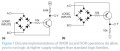# XNOR Logic Gate of Diode Bridge and BJT

#### Electrozapper

Joined Dec 9, 2015
15
The enclosed attachment is the circuit I have some questions about:I see this as an opportunity to learn some things about electronics that heretofore I have somehow missed out on. For one thing, the negative rail is omitted (probably understood but I don't understand it). So, where is the negative rail to (a) and the positive rail to (b)? That is my first question. My second question has to do with reading schematics. My reading of texts and my college work on the subject did not include this data (this part of the education). Therefore, I must from this site learn how such data can be supplied, filled in, understood, what have you? Can you make a reference or simply explain how such omitted data can be gleaned? My last question is the assumed switching of Input A and B here. Could someone draw that in, as well? I assume (for sake of example) that it is like so many other lesson types: a double pole, single throw. Still, how is that filled in to the schematic to make a complete circuit? Thanks ahead of time!

#### MikeML

Joined Oct 2, 2009
5,444
Last edited:
•Electrozapper

#### GopherT

Joined Nov 23, 2012
8,009
The enclosed attachment is the circuit I have some questions about:

View attachment 96487

I see this as an opportunity to learn some things about electronics that heretofore I have somehow missed out on. For one thing, the negative rail is omitted (probably understood but I don't understand it). So, where is the negative rail to (a) and the positive rail to (b)? That is my first question. My second question has to do with reading schematics. My reading of texts and my college work on the subject did not include this data (this part of the education). Therefore, I must from this site learn how such data can be supplied, filled in, understood, what have you? Can you make a reference or simply explain how such omitted data can be gleaned? My last question is the assumed switching of Input A and B here. Could someone draw that in, as well? I assume (for sake of example) that it is like so many other lesson types: a double pole, single throw. Still, how is that filled in to the schematic to make a complete circuit? Thanks ahead of time!
Only the positive rail is shown for (a) and only ground is shown in the circuit on (b) because the connection from the missing rail is actually coming from the input. The input is either at ground (0V) or at supply voltage (Vcc).

V(a) and V(b) are driven from perfect voltage sources that produce either 5V or 0V. I will leave it as an exercise to the student as to why V(o) is not driven all the way to 0V.

View attachment 96491
@MikeML

The discrete version is used to avoid limitations on max voltage. The voltage can be anything up to the limits of the transistor, diodes or current limit of the resistors at supply voltage levels.

#### crutschow

Joined Mar 14, 2008
32,059
...............
I see this as an opportunity to learn some things about electronics that heretofore I have somehow missed out on. For one thing, the negative rail is omitted (probably understood but I don't understand it). So, where is the negative rail to (a) and the positive rail to (b)?
....................
The negative rail (ground) is implied in (a) as the reference for the source voltage for inputs A and B and also is the reference for the (+) voltage and the Y output voltage.

There is no positive rail for (b).
Inputs A and B are referenced to ground and provide the power to the circuit.

•Electrozapper